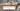# Swap Nodes Pairs in Link List - Leet Code Solution

September 16, 2019## Problem Statement

Given a linked list, swap every two adjacent nodes and return its head. You may not modify the values in the list’s nodes, only nodes itself may be changed.

``````Example:

Given 1->2->3->4, you should return the list as 2->1->4->3.``````

## Solution

• Lets look at the first two nodes first.
• We need to move head to second. (One time operation)
• Move next of First to next of Second
• Move next of Second to First
• All looks fine. We can go like this in pairs.
• Now, what about joining the pairs?
• We need another pointer who will be responsible of joining these pairs.

## Code

``````public static class ListNode {
int val;
ListNode next;
ListNode(int x) { val = x; }
@Override
public String toString() {
StringBuffer sb = new StringBuffer();
ListNode t = this;
while (t != null) {
sb.append(t.val).append(" -> ");
t = t.next;
}
return sb.toString();
}
}

ListNode prev = null;

ListNode result = second;
while (first != null && second != null) {
first.next = second.next;
second.next = first;

if (prev != null) {
prev.next = second;
}

prev = first;

first = first.next;
if (first != null) {
second = first.next;
}
}

return result;
}``````

## Leet Code submission result

Runtime: 0 ms, faster than 100.00% of Java online submissions for Swap Nodes in Pairs. Memory Usage: 34.5 MB, less than 100.00% of Java online submissions for Swap Nodes in Pairs.